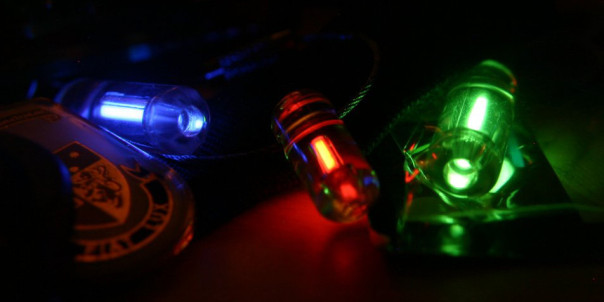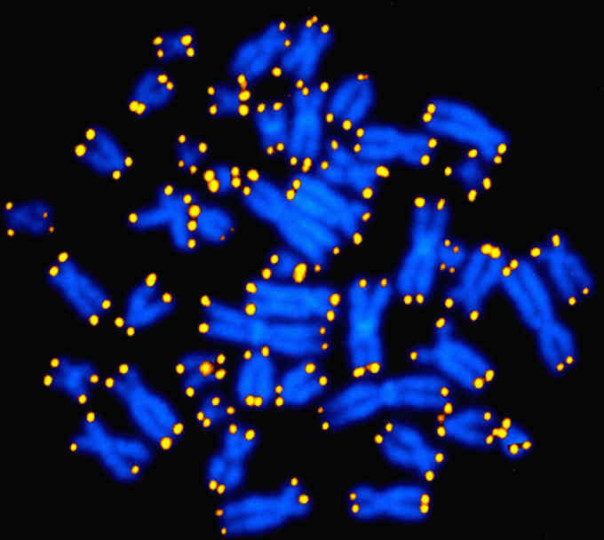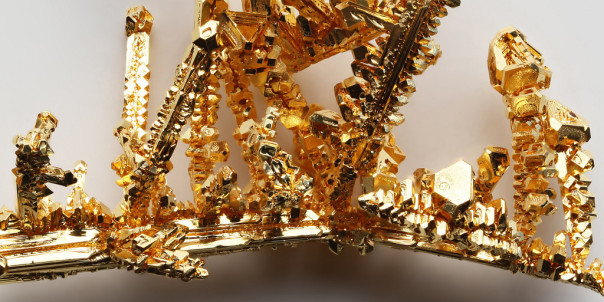# Tritium Illumination

Radioluminesence is the emission of light due to bombardment by ionising radiation, the most common example of which is tritium illumination. Tritium is an isotope of hydrogen, made up of one proton and two neutrons (i.e. it is hydrogen-3). It has a half-life of 12.3 years and decays by emitting beta particles (high speed electrons) to form helium-3.

In a tritium illumination light source the tritium gas is trapped in a glass tube that has been coated on the inside with a phosphor. When the gas decays, the electrons produced strike the phosphor and their kinetic energy is transferred into light energy. By choosing different phosphors, different colours of light are produced.Novelty keychains containing tritium light sources.

Because tritium illumination requires no power source and lasts for a long time, it is commonly used in situations where long-term but low-power lighting is required. For example, tritium illumination is used on watchfaces, compasses, instrument dials and gunsights. The encapsulation of the tritium source prevents any radiation risk, and if a tritium light source is broken open then simply leaving the area and allowing the gas to disperse will mitigate any health risks.

# Telomeres

A telomere is a section of repetitive DNA* found at the end of the chromatids that make up a chromosome. Unlike normal DNA, telomeres do not code for the production of proteins – they are non-coding DNA. The purpose of telomeres is to protect the important coding DNA during the DNA copying process; without telomeres the ends of each chromatid would be lost during cell division. Dr Elizabeth Blackburn, who won the 2009 Nobel Prize in Medicine or Physiology for her work on telomeres likened them to the plastic caps on the ends of shoelaces that prevent them from fraying.Human chromosomes with telomeres highlighted in yellow.

Imagine that cell division works like photocopying, with each division making a perfect photocopy of the original. However, this photocopier is not perfect, and always loses the last five pages in the copying process, making the copy five pages shorter than the original. Adding telomeres to the end of chromatids is like adding many blank pages to the end of your original before the copying process starts. Although five pages are lost during each copy, there are many, many blank pages that can afford to be lost before important (i.e. coding DNA) pages begin to be lost.

During the aging process telomeres become shorter and shorter, and the shorter your telomeres are the more susceptible you are to disease and the shorter your lifespan is likely to be.

* In humans telomeres code the sequence “TTAGGG” over and over again.

# Chrysopoeia

Chrysopoeia is the artificial production of gold, long the goal of alchemists (particularly the transmutation of base metals, such as lead). Turning one element into another via chemical reactions is impossible, but it is possible using nuclear reactions.Crystalline gold

Gold has only one stable isotope, gold-197, so any nuclear reaction aiming to produce gold must finish with producing gold-197. If your reaction produces another gold isotope, such as gold-196 or gold-198 this will decay over the course of a few days to form another element (platinum-196 or mercury-196 in the case of gold-196, and mercury-198 in the case of gold-198).

Producing gold from lead is impossible, but it is possible to turn mercury into gold. If mercury-196 (0.15% of natural mercury) is irradiated with slow neutrons it forms mercury-197 which then decays via electron capture to form stable gold-197. If mercury-198 (9.97% of natural mercury) is used instead, it can be irradiated with fast neutrons, causing it to lose a neutron and form mercury-197 and then gold-197 as above.

In 1980 Glenn Seaborg (after whom seaborgium is named) produced a tiny amount of gold (thousands of atoms) by bombarding bismuth-209 with carbon-12 and neon-20 atoms, but only formed radioactive isotopes of gold in the process.

Regardless of the method used, the production of gold in nuclear reactions is prohibitively expensive, costing many times the price of gold per unit mass produced.

# Averages

An average is way of expressing, in a single figure, important information about a population.

The arithmetic mean is probably what you think of when you think of average. To find the arithmetic mean you sum all the values in your set, and then divide by the number of values. So the arithmetic mean of 1, 1, 2, 3, 5, and 8 is 20/6 or 3⅓.

The median is the middle value within a set when the set is arranged in order. So the median of 1, 1, 2, 3, 5, 8, 13 is 3, because 3 is the fourth value in a set of seven values. If the number of values in the set is even, then the median is half-way between the two middle values. Therefore the median of  1, 1, 2, 3, 5, 8 is 2.5, because 2 and 3 are the third and fourth value in a set of six values.

The median is useful when your data contains outliers. For example, in a class of ten pupils who score 91%, 92%, 93%, 94%, 95%, 96%, 96%, 98%, 99% and 10% the arithmetic mean average is 86.5%. Does this seem correct? Would it be correct to report this as the class’s “average mark”? In this situation it’s more sensible to report the median mark, which in this case is 95.5%.

The median is the most resistant average – it takes a great deal of contamination (e.g. by outlier values) to cause it to breakdown and give an arbitrarily large or small value. To corrupt the median value, more than 50% of the data have to be “contaminated”, in which case your data-collection process is probably fundamentally flawed.

The mode is the most common value within a set. So the mode of 1, 1, 2, 3, 5, 8 is 1, because 1 appears twice and the rest of the numbers only appear once. The mode is the only average that makes sense when dealing with non-numerical data: the mode eye colour (brown in the UK), or the mode surname (Smith in the UK), for example.

The geometric mean is useful when you are comparing values that have different ranges. For example, take the two computers specified below:

 CompuTron 9001 Comp-O-Matic A1 Clock Speed /GHz 4.00 4.50 RAM /GB 4.00 8.00 Hard Disk /GB 1250 1000 Arithmetic Mean 419 338 Geometric Mean 27.1 33.0

The CompuTron 9001 scores higher on the arithmetic mean because the size of the hard disk has a disproportionate effect (it is of the order of 103, whereas the clock speed and RAM values are of the order of 100), but the geometric mean shows that the Comp-O-Matic A1 is better overall.

The geometric mean of a set of n values is the nth-root of the product of the values in the set, or in algebraic terms:$\bar{x}_{GM}=\left(\prod_{i=1}^n{x_i}\right)^{\frac{1}{n}}$

The geometric mean is also useful when your data has a very large range. For example, if we looked at the gross domestic product (GDP) of ten countries picked at random we might end up with the data shown below:

 Country GDP /$bn Country GDP /$bn Slovenia 50.3 Spain 1480 Niger 6.38 Ukraine 165 USA 15000 Bermuda 5.97 Albania 13.0 Jordan 28.8 Monaco 5.92 Croatia 62.5

Here the largest value (USA) is more than two-and-a-half thousand times larger than the smallest value (Monaco). Is it fair to say that the “average” GDP for countries in this list is the arithmetic mean of $1680 billion, when nine out of the ten countries in the list have a GDP less than this, and seven of the ten have a GDP less than one-tenth of this? For these countries the geometric mean of$62.9 billion might be a better choice. (The median is probably not a good choice as we have a very limited data set with a long tail.)

The harmonic mean is especially important in physics, particularly when dealing with rates (e.g. speed, acceleration) and ratios (e.g. resistance, capacitance). If a car drives 100 kilometres one way at 60 km/h and then back the same distance at 40 km/h you would be forgiven for thinking that its “average” speed is 50 km/h. However, this is not true as it doesn’t take account of the fact that the car spends more time at 40 km/h than it does at 60 km/h.

Calculating the harmonic mean of these two speeds using the equation below yields the correct average speed of 48 km/h.$\bar{x}_{HM}=\left(\frac{1}{n}\sum_{i=1}^n x_{i}^{-1}\right)^{-1}$

The same is true when considering fuel economy: the average miles per gallon figure for two cars, one 30 mpg and one 50 mpg driving the same distance is not 40 mpg but rather the harmonic mean of the two figures, 37.5 mpg.

In a network of n resistors in parallel, or n capacitors in series, the harmonic mean of the resistors’ or capacitors’ values yields the correct average value of each resistor’s or capacitor’s contribution to the network. For example: a 90Ω and 10Ω resistor in parallel have a combined resistance of 9Ω. The harmonic mean of 90Ω and 10Ω is 18Ω, and two 18Ω resistors in parallel yield a total resistance of 9Ω. (If the resistors are in series, or the capacitors in parallel, then the arithmetic mean should be used.)

The weighted mean is similar to the arithmetic mean, but takes account of the relative contributions of each component. Consider the data below:

 Subject Number of Students Pass Rate Science 100 100% English 400 50% Mathematics 400 50%

A naïve Headteacher might simply take the average of 100%, 50% and 50% and claim that the overall pass rate was 68%. However, this fails to take account of the fact that far more students were studying English and Maths than were studying Science, and so the correct average pass rate was 56%.

There is not necessarily a “correct” average to use for any given situation. You should base your choice of average on trying to fulfil the criterion at the top of this post: a single number that best represents the entire set of data.